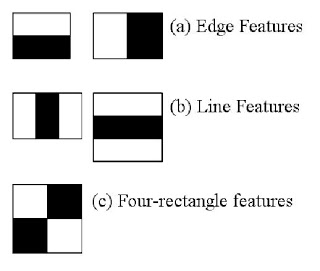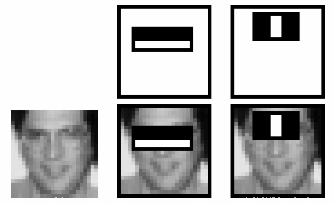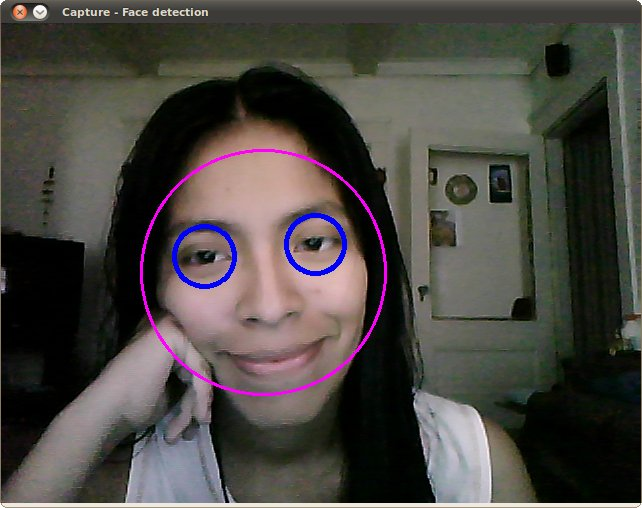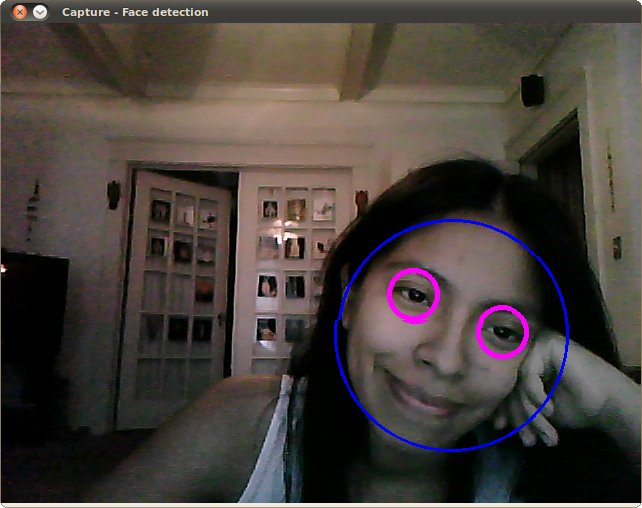# 级联分类器¶

### 理论¶### OpenCV中的Haar-级联检测器¶

from __future__ import print_function
import cv2 as cv
import argparse
def detectAndDisplay(frame):
frame_gray = cv.cvtColor(frame, cv.COLOR_BGR2GRAY)
frame_gray = cv.equalizeHist(frame_gray)
#-- 检测面部
for (x,y,w,h) in faces:
center = (x + w//2, y + h//2)
frame = cv.ellipse(frame, center, (w//2, h//2), 0, 0, 360, (255, 0, 255), 4)
faceROI = frame_gray[y:y+h,x:x+w]
#-- 在每张面部上检测眼睛
for (x2,y2,w2,h2) in eyes:
eye_center = (x + x2 + w2//2, y + y2 + h2//2)
radius = int(round((w2 + h2)*0.25))
frame = cv.circle(frame, eye_center, radius, (255, 0, 0 ), 4)
cv.imshow('Capture - Face detection', frame)
parser = argparse.ArgumentParser(description='Code for Cascade Classifier tutorial.')
parser.add_argument('--camera', help='Camera divide number.', type=int, default=0)
args = parser.parse_args()
#-- 1. 加载级联
exit(0)
exit(0)
camera_device = args.camera
#-- 2. 读取视频流
cap = cv.VideoCapture(camera_device)
if not cap.isOpened:
print('--(!)Error opening video capture')
exit(0)
while True:
ret, frame = cap.read()
if frame is None:
print('--(!) No captured frame -- Break!')
break
detectAndDisplay(frame)
if cv.waitKey(10) == 27:
break